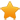Switch Editions?
CancelChannel: CodeSection,代码区,网络安全 - CodeSec
Viewing all articles

# Using emojis as scatterplot points

0
0

(This article was first published on R Statistical Odds & Ends , and kindly contributed toR-bloggers)

Recently I wanted to learn how to use emojis as points in a scatterplot points. It seems like the emojifont package is a popular way to do it. However, I couldn’t seem to get it to work on my machine (perhaps I need to install the font manually?). The other package I found was emoGG ; this post shows how to use this package. (For another example involving fire stations, see this script .)

Let’s load the packages:

library(ggplot2)
library(emoGG)

Let’s load a less commonly used small dataset that comes with base R called ToothGrowth :

data("ToothGrowth")
summary(ToothGrowth)
# len supp dose
# Min. : 4.20 OJ:30 Min. :0.500
# 1st Qu.:13.07 VC:30 1st Qu.:0.500
# Median :19.25 Median :1.000
# Mean :18.81 Mean :1.167
# 3rd Qu.:25.27 3rd Qu.:2.000
# Max. :33.90 Max. :2.000

Here is the dataset’s description from its documentation:

The response is the length of odontoblasts (cells responsible for tooth growth) in 60 guinea pigs. Each animal received one of three dose levels of vitamin C (0.5, 1, and 2 mg/day) by one of two delivery methods, orange juice or ascorbic acid (a form of vitamin C and coded as VC ).

A little esoteric… but it’ll work for our purposes. Let’s make a scatterplot of len vs. dose :

ggplot(data = ToothGrowth) +
geom_point(aes(dose, len))

To plot emojis instead of points, we use the geom_emoji layer and specify which emoji to use with the emoji option:

ggplot(data = ToothGrowth) +
geom_emoji(aes(dose, len), emoji = "1f439") +
labs(x = "Dose (mg/day)", y = "Tooth length")

We can add horizontal jitter to the points manually:

ggplot(data = ToothGrowth) +
geom_emoji(aes(dose + runif(nrow(ToothGrowth), min = -0.2, max = 0.2),
len), emoji = "1f439") +
labs(x = "Dose (mg/day)", y = "Tooth length")

I wasn’t able to find an elegant way to have different emojis printed for different points. For example, this dataset contains 30 guinea pigs fed by orange juice and 30 fed by ascorbic acid: we might want different emojis to represent these two groups. The following code does not work:

# doesn't work
ggplot(data = ToothGrowth) +
geom_emoji(aes(dose + runif(nrow(ToothGrowth), min = -0.2, max = 0.2),
len), emoji = supp) +
labs(x = "Dose (mg/day)", y = "Tooth length")

What we could do is add separate layers manually for each of the 30 guinea pigs. It’s cumbersome but it works…

p1 <- geom_emoji(data = subset(ToothGrowth, supp == "OJ"),
aes(dose + runif(sum(ToothGrowth\$supp == "OJ"), min = -0.2, max = 0.2),
len), emoji = "1f34a")
p2 <- geom_emoji(data = subset(ToothGrowth, supp == "VC"),
aes(dose + runif(sum(ToothGrowth\$supp == "OJ"), min = -0.2, max = 0.2),
len), emoji = "1f48a")
ggplot() +
p1 + p2 +
labs(x = "Dose (mg/day)", y = "Tooth length")

How did I find the emoji code to use? emoGG comes with an emoji_search function that helps us with that. For example, to find the code for the orange emoji:

emoji_search("orange")
# emoji code keyword
# 2184 tangerine 1f34a orange
# 2235 carrot 1f955 orange
# 4092 a 1f170 red-square
# 4093 a 1f170 alphabet
# 4094 a 1f170 letter
# 4130 o 2b55 circle
# 4131 o 2b55 round
# 4364 ng 1f196 blue-square
# 4365 ng 1f196 words
# 4366 ng 1f196 shape
# 4367 ng 1f196 icon
# 5311 georgia 1f1ec\\U0001f1ea ge

Viewing all articles

More Pages to Explore .....